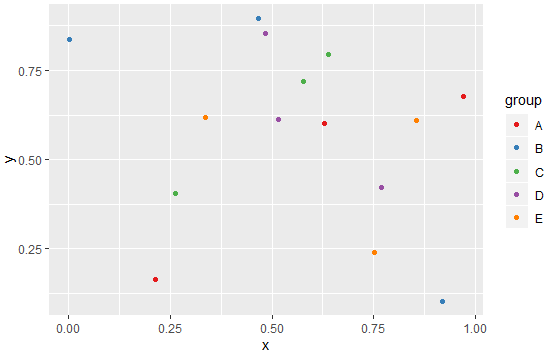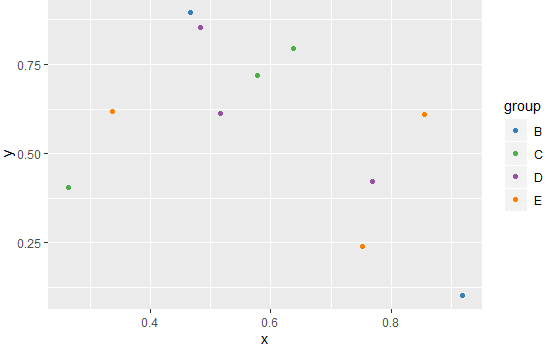2 views

I've been getting up to speed with R in the last month.

Here is my question:

What is a good way to assign colors to categorical variables in ggplot2 that have stable mapping? I need consistent colors across a set of graphs that have different subsets and a different number of categorical variables.

For example,

plot1 <- ggplot(data, aes(xData, yData,color=categoricaldData)) + geom_line()

where categoricalData has 5 levels.

And then

plot2 <- ggplot(data.subset, aes(xData.subset, yData.subset,

color=categoricaldData.subset)) + geom_line()

where categoricalData.subset has 3 levels.

However, a particular level that is in both sets will end up with a different color, which makes it harder to read the graphs together.

Do I need to create a vector of colors in the data frame? Or is there another way to assigns specific colors to categories?

by

To assign colors to categorical variables in ggplot2, you can create a color scale manually and assign the colors to the categorical values as follows:

To create a sample data frame:

df <- data.frame(x=runif(15),y=runif(15),

group = rep(LETTERS[1:5],each = 3),stringsAsFactors = TRUE)

To create a custom color scale:

library(RColorBrewer)

myColorScale <- brewer.pal(5,"Set1")

names(myColorScale) <- levels(df\$group)

colScale <- scale_colour_manual(name = "group",values = myColors)

To plot the data with all categorical values:

p <- ggplot(df,aes(x,y,colour = group)) + geom_point()

p1 <- p + colScale

p1

Output:To plot with only four levels:

p2 <- p %+% droplevels(subset(df[5:15,])) + colScale

p2

Output: